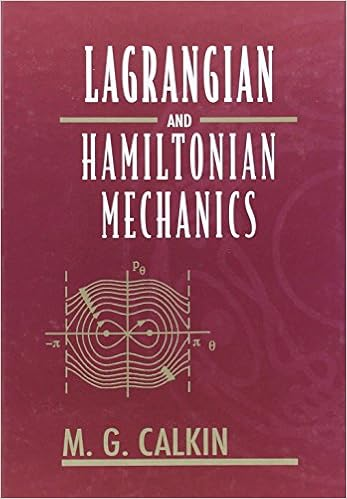## Wednesday, September 16, 2015

### Lagrangian and Hamiltonian Mechanics by M. G. CalkinThis book takes the student from the Newtonian mechanics typically taught in the first and the second year to the areas of recent research. The discussion of topics such as invariance, Hamiltonian Jacobi theory, and action-angle variables is especially complete; the last includes a discussion of the Hannay angle, not found in other texts. The final chapter is an introduction to the dynamics of nonlinear nondissipative systems. Connections with other areas of physics which the student is likely to be studying at the same time, such as electromagnetism and quantum mechanics, are made where possible. There is thus a discussion of electromagnetic field momentum and mechanical "hidden"; momentum in the quasi-static interaction of an electric charge and a magnet. This discussion, among other things explains the "(e/c)A"; term in the canonical momentum of a charged particle in an electromagnetic field. There is also a brief introduction to path integrals and their connection with Hamilton's principle, and the relation between the Hamilton Jacobi equation of mechanics, the eikonal equation of optics, and the Schrödinger equation of quantum mechanics. The text contains 115 exercises. This text is suitable for a course in classical mechanics at the advanced undergraduate level.